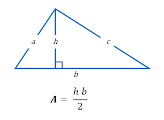## C program to find area of a triangle

Write a C program to input base and height of a triangle and find area of the given triangle. How to find area of a triangle in C programming. Logic to find area of a triangle in C program.

#### Required knowledge

Operators, Data Types in c, Variables in C, Basic input/output

#### Area of a triangle

Area of a triangle is given by formula.Where b is base and h is height of the triangle.

#### Logic to find area of a triangle

Below is the step by step descriptive logic to find area of a triangle.

1. Input base of the triangle. Store in some variable say base.

2. Input height of the triangle. Store in some variable say height.

3. Use triangle area formula to calculate area i.e. area = (base * height) / 2.

4. Print the resultant value of area.

#### Program to find area of a triangle

```
/**
* C program to find area of a triangle if base and height are given
*/

#include <stdio.h>

int main()
{
float base, height, area;

/* Input base and height of triangle */
printf("Enter base of the triangle: ");
scanf("%f", &base);
printf("Enter height of the triangle: ");
scanf("%f", &height);

/* Calculate area of triangle */
area = (base * height) / 2;

/* Print the resultant area */
printf("Area of the triangle = %.2f sq. units", area);

return 0;
}
```

%.2f is used to print fractional values only up to 2 decimal places. You can also use %f to print up to 6 decimal places by default.

##### Output:
```
Enter base of the triangle: 10
Enter height of the triangle: 15
Area of the triangle = 75.00 sq. units
```
```
Recommended posts

Loop programming exercises index.
Basic programming exercises index.
If else programming exercises index.
Array programming exercises index.
String programming exercises index.
Pointer programming exercises index.
Star Patterns exercises index.
Number Patterns exercises index.
alphabet Patterns exercises index.
series program exercises index.

```